# Construction Of Anderson’s Bridge Circuit, Its Working And Application

The bridge circuits are used to measure various component values like resistance, capacitance, inductance, etc. The simple form of a bridge circuit consists of a network of four resistances/impedance arms that forms a closed circuit. A current source is applied to two opposite nodes and a current detector is connected to the remaining two nodes. This article discusses the Andersons bridge circuit working and its applications.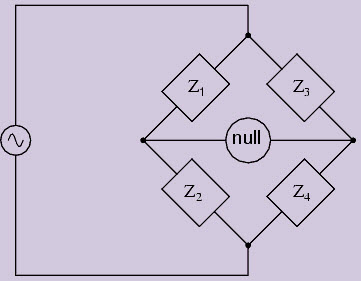The bridge circuits use the null indication principle and comparison measurement method, this is also known as “Bridge Balance condition at zero voltage. The bridge circuit compares the values of an unknown component with that of an accurately known standard component. Thus, the accuracy mostly depends on the bridge circuit, not on the null-indicator.

From the above bridge circuit, the balancing equation is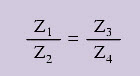## Different Types of Bridges

Two types of bridges used to measure the component values. They are D.C bridges and A.C bridges.

D.C Bridges are

The various types of A.C Bridges are,

• Inductance comparison bridge
• Capacitance comparison bridge
• Maxwell’s bridge
• Hay’s bridge
• Anderson’s bridge
• Schering bridge
• Wien bridge

#### A.C Bridges

AC bridges are often used to measure the value of the unknown impedance (self/mutual inductance of inductors or capacitance of capacitors accurately). An A.C bridge circuit consists of four impedances, a source of A.C supply and a balanced detector. The balance detectors generally used for A.C bridges are

• Headphones (at the frequencies of 250 Hz to 3 to 4 kHz)
• Tunable amplifier circuit (for a frequency range of 10HZ to 100Hz)
• Vibration galvanometers (for low range frequency from 5Hz to 1000 Hz)

The null response (bridge balance condition) can be obtained by varying one of the bridge arms. The impedance of a component is in a form of polar that can have a magnitude and a phase angle value. For an A.C circuit shown above, the impedance can be written in terms of magnitude and phase angle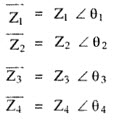Where Z1, Z2, Z3, Z4, are the magnitudes and θ1, θ2, θ3, and θ4 are phase angles. The product of all impedances must be carried out in the polar form where all magnitudes get multiplied and phase angles should be added.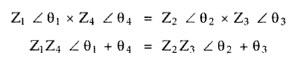Here, the bridge must be balanced for both the conditions magnitude as well as phase angles. From the above equations two conditions to be satisfied for the bridge balance. Equating magnitudes of both sides, we will get the magnitude condition as,

Z1.Z4=Z2.Z3

And the phase angles also, θ1+ θ4= θ2+ θ3

The phase angle is +ve inductive impedances and –ve for the capacitive impedances.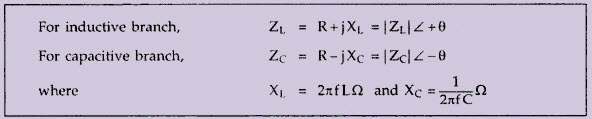## Andersons Bridge Construction and Working

The Anderson’s Bridge is an A.C bridge used to measure the self-inductance of the coil. It enables to measure the inductance of a coil using a standard capacitor and resistors. It does not require a repeated balancing of the bridge. It is a modification of Maxwell’s Bridge in which also the value of self-inductance is obtained by comparing it with a standard capacitor. The connections are shown below.

One arm of the bridge consists of the unknown inductor Lx with known resistance in series with Lx. This resistance R1 includes the resistance of the inductor. Capacitance C is the standard capacitor with r, R2, R3, and R4 are non-inductive in nature.

The bridge balance equations are,

i1= i3, and i2= i4+ic,

V2= i2.R3 and V3= i3.R3

V1= V2+ic.r and V4= V3+ icr

V1=i1.R1+i1.ω.L1 and V4=i4.R4

Now the voltage V is given by,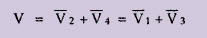From the above circuit, R2, R4, and rare in star form, that is transformed into its equivalent delta form in order to find the bridge balance equations as shown in the figure below.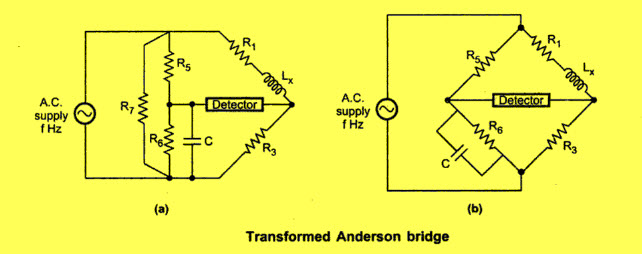The elements in the equivalent delta are given by,

R5= (R2.r+R4.r+R2.R4)/R4

R6= (R2.r+R4.r+R2.R4)/R2

R7= (R2.r+R4.r+R2.R4)/r

Now the R7 shunts the source, and hence it does not affect the balance condition. Thus, by neglecting R7 and rearranging a network as above fig (b), we get a Maxwell inductance bridge.

Thus the balance equation is given by

Lx= CR3R5 and

R1=R3.(R5/R6)

Substituting the values of R5 and R6, we will get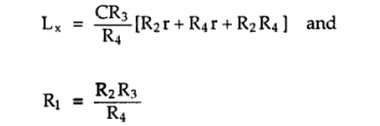If the capacitor used is not perfect, the value of inductance remains unchanged, but the value of R1 changes. The Anderson’s bridge method can also be used to measure of the capacitor C if a calibrated self-inductance is available.

The above equation which we obtained is more complex than we have obtained in the Maxwell bridge. On observing the above equations we can easily say that to obtain convergence of balance more easily, one should make alternate adjustments of R1 and r in Anderson’s bridge.

Now let us look how we can obtain the value of the unknown inductor experimentally. At first, set the signal generator frequency at the audible range. Now adjust R1 and r such that the headphones (null detector) give a minimum sound. Measure the values of R1 and r (obtained after these adjustments) with the help of the multimeter. Use the formula that we have derived above in order to find out the value of unknown inductance. The experiment can be repeated with the different value of the standard capacitor.

• The fixed capacitor is used, whereas other bridges use a variable capacitor.
• The bridge is used for accurate determination of inductance in the millimeter range.
• This bridge also gives an accurate result for the determination of capacitance in terms of inductance.
• The bridge is easy to balance from the convergence point of view compared to Maxwell’s bridge in case of low values of Q.